Test: Queues

# Test: Queues

Test Description

## 15 Questions MCQ Test | Test: Queues

Test: Queues for Computer Science Engineering (CSE) 2023 is part of Computer Science Engineering (CSE) preparation. The Test: Queues questions and answers have been prepared according to the Computer Science Engineering (CSE) exam syllabus.The Test: Queues MCQs are made for Computer Science Engineering (CSE) 2023 Exam. Find important definitions, questions, notes, meanings, examples, exercises, MCQs and online tests for Test: Queues below.
Solutions of Test: Queues questions in English are available as part of our course for Computer Science Engineering (CSE) & Test: Queues solutions in Hindi for Computer Science Engineering (CSE) course. Download more important topics, notes, lectures and mock test series for Computer Science Engineering (CSE) Exam by signing up for free. Attempt Test: Queues | 15 questions in 45 minutes | Mock test for Computer Science Engineering (CSE) preparation | Free important questions MCQ to study for Computer Science Engineering (CSE) Exam | Download free PDF with solutions
 1 Crore+ students have signed up on EduRev. Have you?
Test: Queues - Question 1

### Following is C like pseudo code of a function that takes a Queue as an argument, and uses a stack S to do processing. void fun(Queue *Q)  {      Stack S; // Say it creates an empty stack S      // Run while Q is not empty      while (!isEmpty(Q))      {          // deQueue an item from Q and push the dequeued item to S          push(&S, deQueue(Q));      }      // Run while Stack S is not empty      while (!isEmpty(&S))      {      // Pop an item from S and enqueue the poppped item to Q      enQueue(Q, pop(&S));      }  }  Q. What does the above function do in general?

Detailed Solution for Test: Queues - Question 1

The function takes a queue Q as an argument. It dequeues all items of Q and pushes them to a stack S. Then pops all items of S and enqueues the items back to Q. Since stack is LIFO order, all items of queue are reversed.

Test: Queues - Question 2

### Which one of the following is an application of Queue Data Structure?

Test: Queues - Question 3

### How many stacks are needed to implement a queue. Consider the situation where no other data structure like arrays, linked list is available to you.

Detailed Solution for Test: Queues - Question 3

A queue can be implemented using two stacks. See following for implementation.

Test: Queues - Question 4

How many queues are needed to implement a stack. Consider the situation where no other data structure like arrays, linked list is available to you.

Detailed Solution for Test: Queues - Question 4

A stack can be implemented using two queues. Please see following for details.

Test: Queues - Question 5

A priority queue can efficiently implemented using which of the following data structures? Assume that the number of insert and peek (operation to see the current highest priority item) and extraction (remove the highest priority item) operations are almost same.

Test: Queues - Question 6

Which of the following is true about linked list implementation of queue?

Detailed Solution for Test: Queues - Question 6

To keep the First IFirst Out order, a queue can be implemented using linked list in any of the given two ways.

Test: Queues - Question 7

Suppose a circular queue of capacity (n – 1) elements is implemented with an array of n elements. Assume that the insertion and deletion operation are carried out using REAR and FRONT as array index variables, respectively. Initially, REAR = FRONT = 0. The conditions to detect queue full and queue empty are

Detailed Solution for Test: Queues - Question 7

Suppose we start filling the queue.

Let the maxQueueSize ( Capacity of the Queue) is 4.
So the size of the array which is used to implement this circular queue is 5, which is n.

In the begining when the queue is empty, FRONT and REAR point to 0 index in the array.

REAR represents insertion at the REAR index.
FRONT represents deletion from the FRONT index.

enqueue("a"); REAR = (REAR+1)%5; ( FRONT = 0, REAR = 1)
enqueue("b"); REAR = (REAR+1)%5; ( FRONT = 0, REAR = 2)
enqueue("c"); REAR = (REAR+1)%5; ( FRONT = 0, REAR = 3)
enqueue("d"); REAR = (REAR+1)%5; ( FRONT = 0, REAR = 4)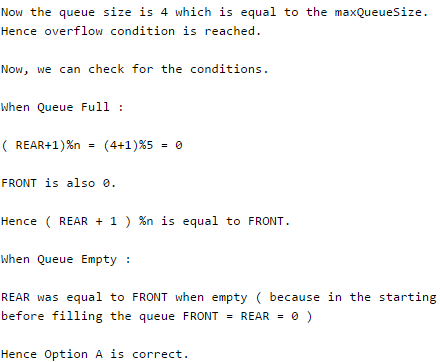Test: Queues - Question 8

A Priority-Queue is implemented as a Max-Heap. Initially, it has 5 elements. The level-order traversal of the heap is given below: 10, 8, 5, 3, 2 Two new elements ”1‘ and ”7‘ are inserted in the heap in that order. The level-order traversal of the heap after the insertion of the elements is:

Test: Queues - Question 9

An implementation of a queue Q, using two stacks S1 and S2, is given below: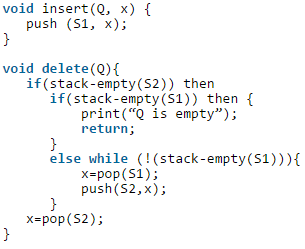Q. Let n insert and m (<=n) delete operations be performed in an arbitrary order on an empty queue Q. Let x and y be the number of push and pop operations performed respectively in the process. Which one of the following is true for all m and n?

Detailed Solution for Test: Queues - Question 9

The order in which insert and delete operations are performed matters here. The best case: Insert and delete operations are performed alternatively. In every delete operation, 2 pop and 1 push operations are performed. So, total m+ n push (n push for insert() and m push for delete()) operations and 2m pop operations are performed. The worst case: First n elements are inserted and then m elements are deleted. In first delete operation, n + 1 pop operations and n push operation are performed. Other than first, in all delete operations, 1 pop operation is performed. So, total m + n pop operations and 2n push operations are performed (n push for insert() and n push for delete())

Test: Queues - Question 10

The following C program is executed on a unix/Linux is: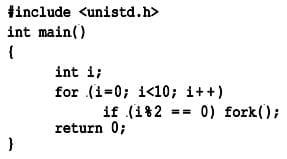The total number of child processes created is ______

Test: Queues - Question 11

Consider the following pseudo code. Assume that IntQueue is an integer queue. What does the function fun do?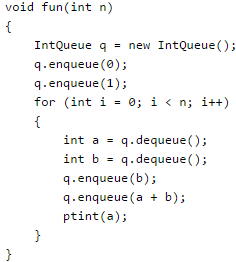Detailed Solution for Test: Queues - Question 11

The function prints first n Fibonacci Numbers. Note that 0 and 1 are initially there in q. In every iteration of loop sum of the two queue items is enqueued and the front item is dequeued.

Test: Queues - Question 12

Consider the following operation along with Enqueue and Dequeue operations on queues, where k is a global parameter.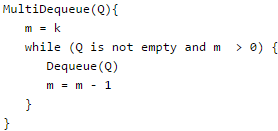Q. What is the worst case time complexity of a sequence of n MultiDequeue() operations on an initially empty queue?

Test: Queues - Question 13

Suppose implementation supports an instruction REVERSE, which reverses the order of elements on the stack, in addition to the PUSH and POP instructions. Which one of the following statements is TRUE with respect to this modified stack?

Detailed Solution for Test: Queues - Question 13

To DEQUEUE an item, simply POP. To ENQUEUE an item, we can do following 3 operations 1) REVERSE 2) PUSH 3) REVERSE

Test: Queues - Question 14

A queue is implemented using an array such that ENQUEUE and DEQUEUE operations are performed efficiently. Which one of the following statements is CORRECT (n refers to the number of items in the queue)?

Detailed Solution for Test: Queues - Question 14

We can use circular array to implement both in O(1) time. See below article for details.

• Queue Introduction and Array Implementation
Test: Queues - Question 15

Let Q denote a queue containing sixteen numbers and S be an empty stack. Head(Q) returns the element at the head of the queue Q without removing it from Q. Similarly Top(S) returns the element at the top of S without removing it from S. Consider the algorithm given below.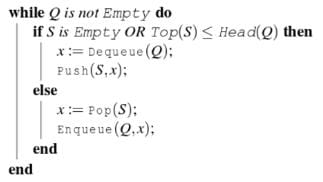Q. The maximum possible number of iterations of the while loop in the algorithm is______
[This Question was originally a Fill-in-the-Blanks question]

Detailed Solution for Test: Queues - Question 15

The worst case happens when the queue is sorted in decreasing order. In worst case, loop runs n*n times.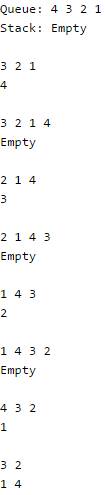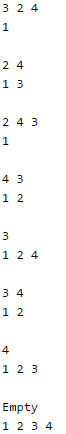(Scan QR code)